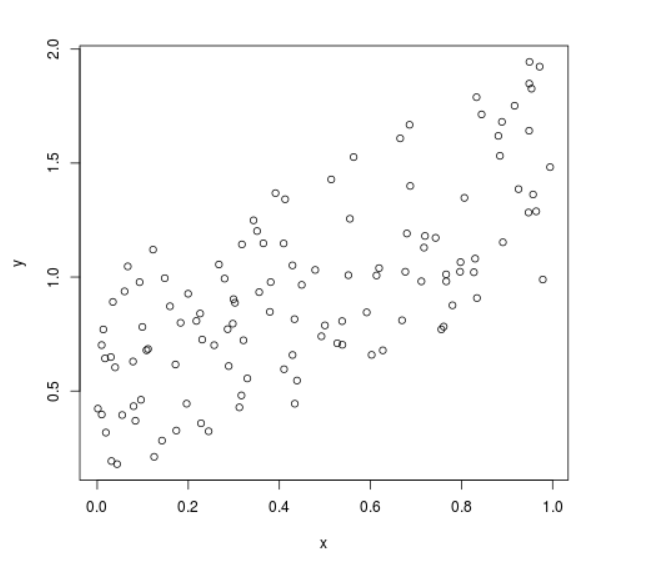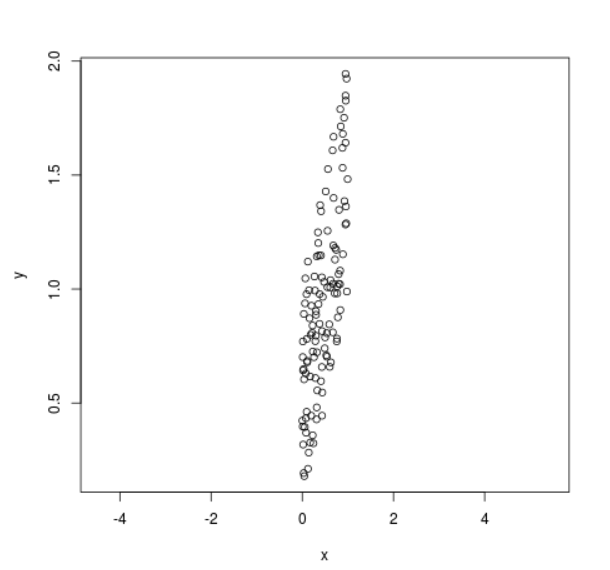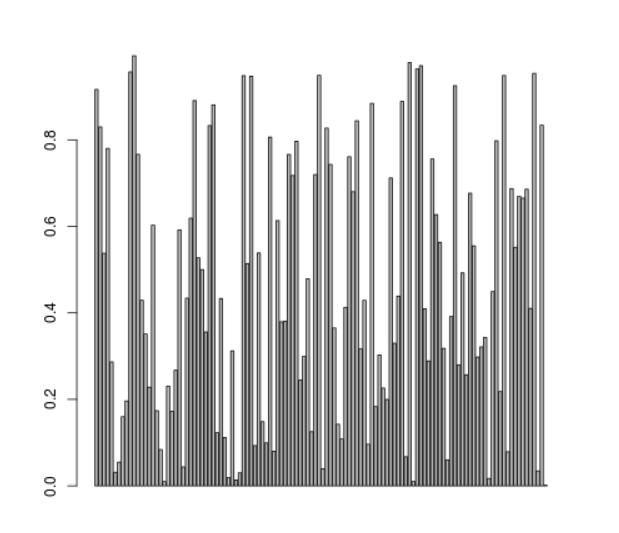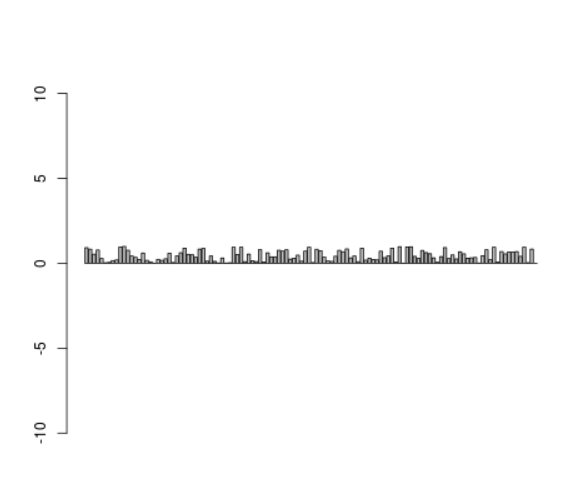# Set Aspect Ratio of Scatter Plot and Bar Plot in R Programming – Using asp in plot() Function

`asp` is a parameter of the `plot()` function in R Language is used to set aspect ratio of plots (Scatterplot and Barplot). Aspect ratio is defined as proportional relationship between width and height of the plot axes.

Syntax: plot(x, y, asp )

Parameters:
x, y: Coordinates of x and y axis
asp: Aspect ratio

Example 1:

 `# Set seed for reproducibility ` `set.seed``(86000)      ` ` `  `# Create random x variable ` `x <- ``runif``(120)      ` ` `  `# Create y variable correlated with x ` `y <- x + ``runif``(120)       ` ` `  `# Plot without setting aspect ratio ` `plot``(x, y)        ` ` `  `# Plot with asp = 5 ` `plot``(x, y, asp = 5)          `

Output:

• Plot Without Aspect Ratio:• Plot with Aspect Ratio:Example 2:

 `# Set seed for reproducibility ` `set.seed``(86000)               ` ` `  `# Create random x variable ` `x <- ``runif``(120)               ` ` `  `# Create y variable correlated with x ` `y <- x + ``runif``(120)  ` ` `  `# Regular barplot ` `barplot``(x)                    ` ` `  `# Barplot with aspect ratio of 5 ` `barplot``(x, asp = 5)          `

Output:

• Bar Plot Without Aspect Ratio:• Bar Plot with Aspect Ratio:Here, the asp option increases the width of the y-axis.

My Personal Notes arrow_drop_upCheck out this Author's contributed articles.

If you like GeeksforGeeks and would like to contribute, you can also write an article using contribute.geeksforgeeks.org or mail your article to contribute@geeksforgeeks.org. See your article appearing on the GeeksforGeeks main page and help other Geeks.

Please Improve this article if you find anything incorrect by clicking on the "Improve Article" button below.

Article Tags :

Be the First to upvote.

Please write to us at contribute@geeksforgeeks.org to report any issue with the above content.